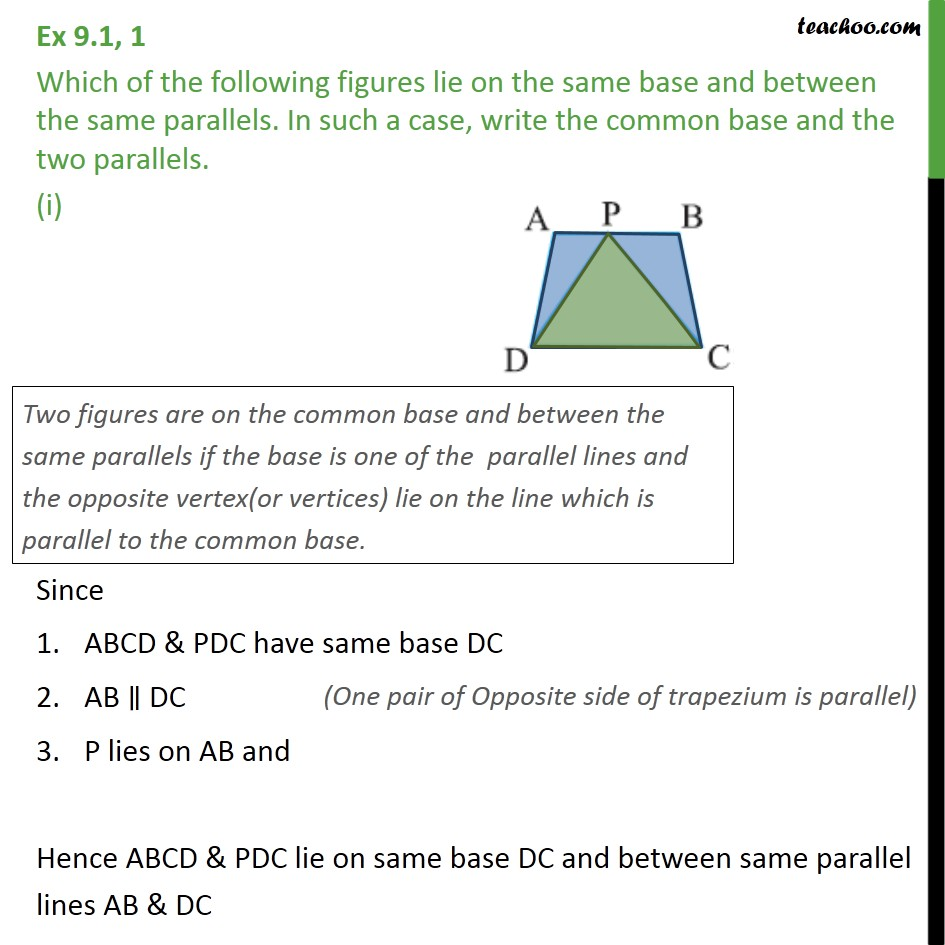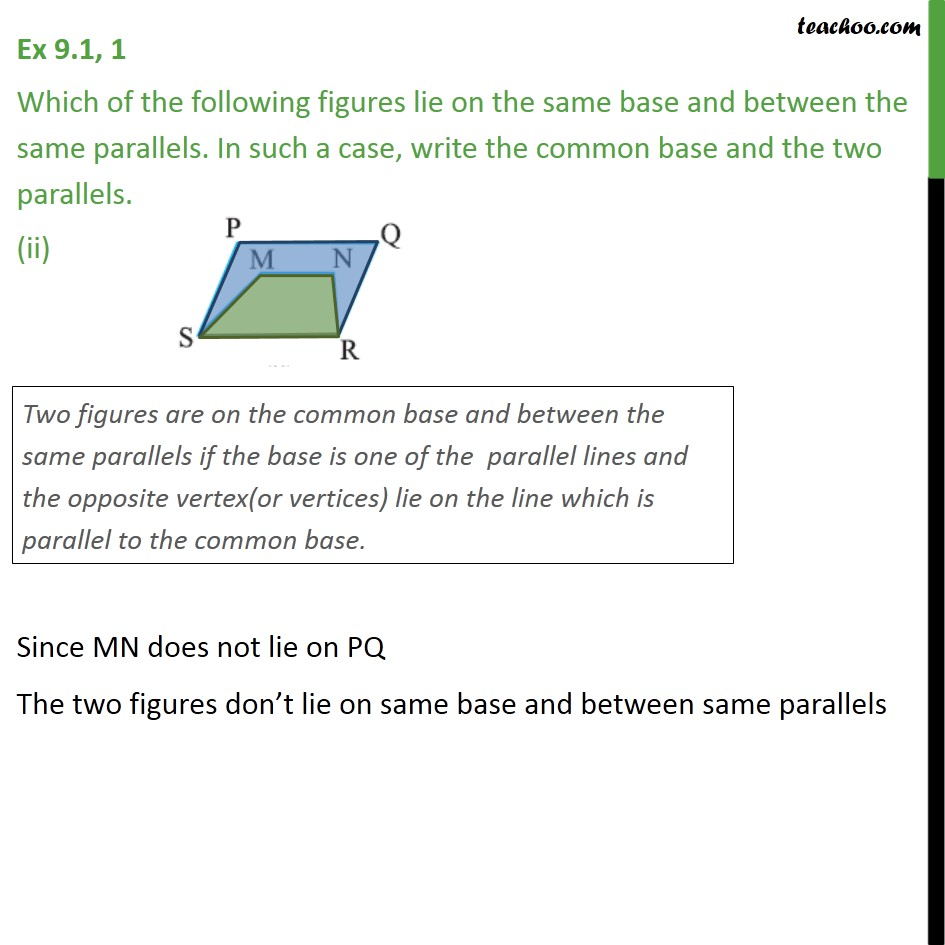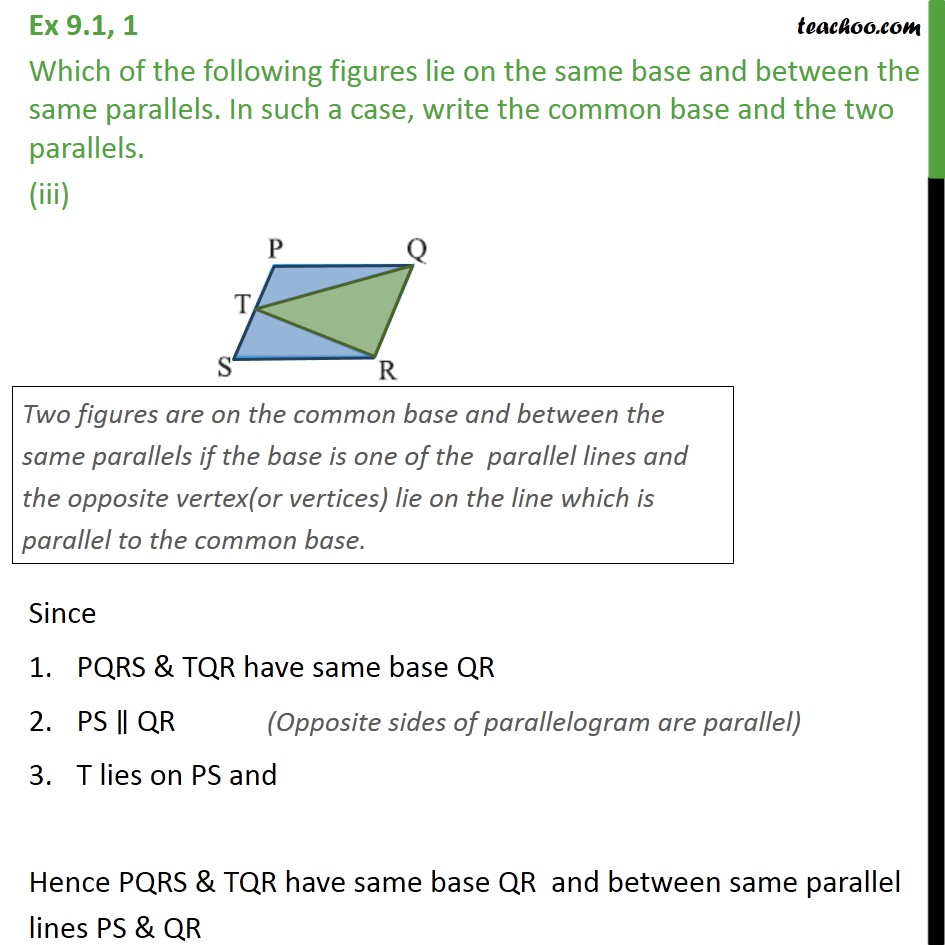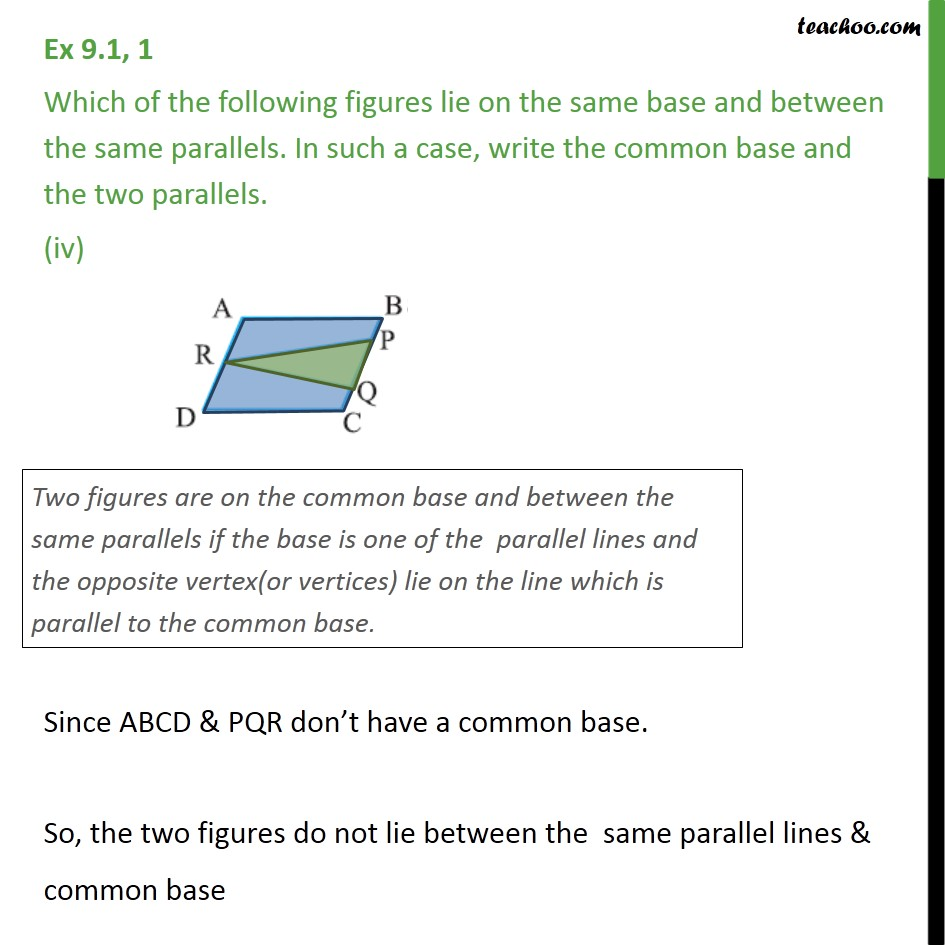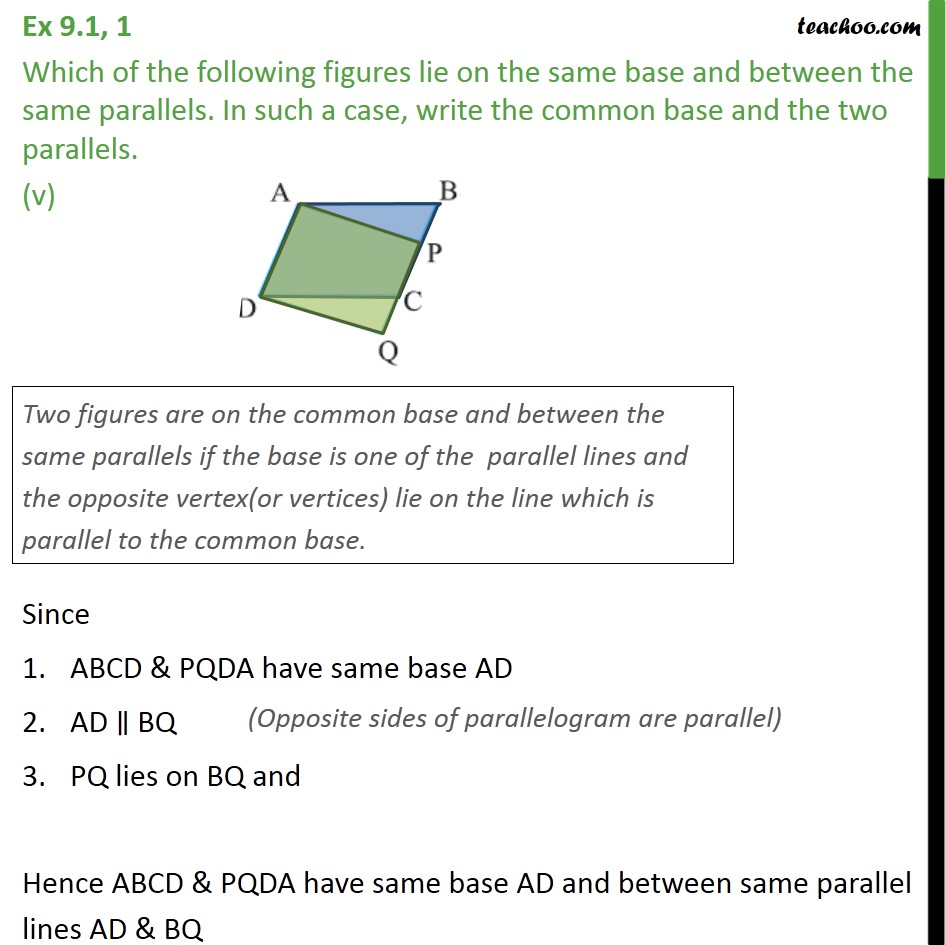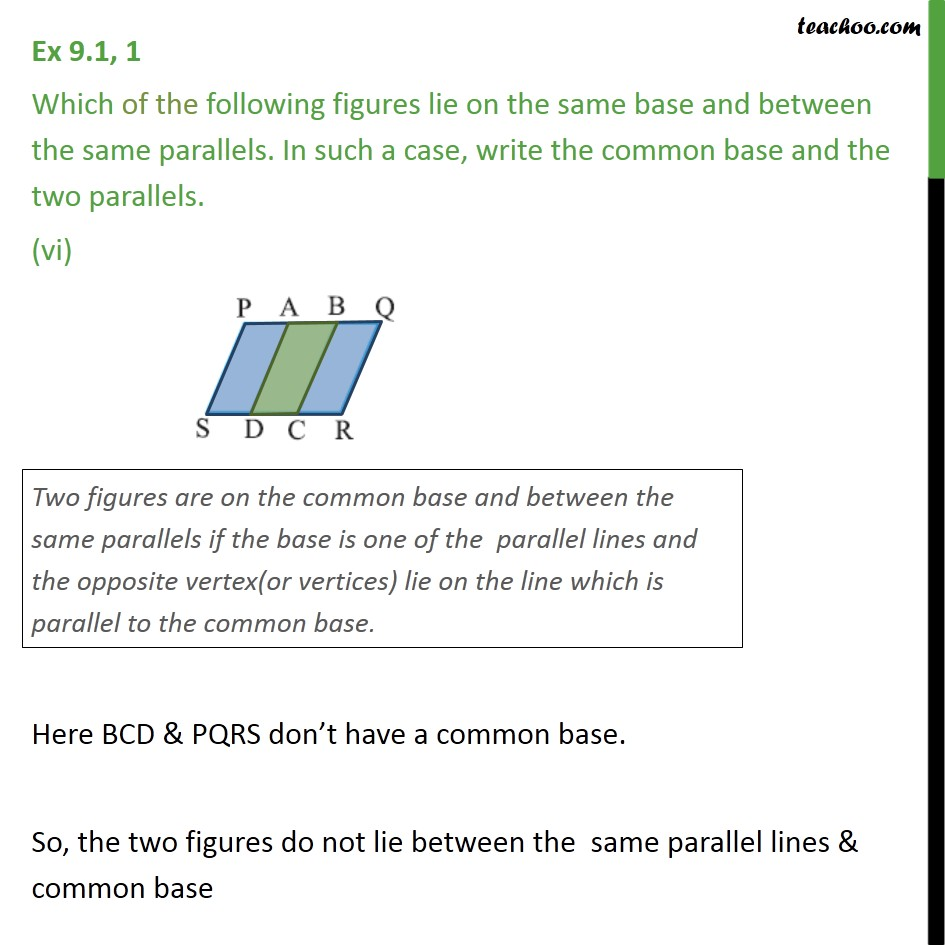Subscribe to our Youtube Channel - https://you.tube/teachoo

1. Chapter 9 Class 9 Areas of Parallelograms and Triangles
2. Concept wise

Transcript

Ex 9.1, 1 Which of the following figures lie on the same base and between the same parallels. In such a case, write the common base and the two parallels. (i) Since ABCD & PDC have same base DC AB ∥ DC P lies on AB and Hence ABCD & PDC lie on same base DC and between same parallel lines AB & DC Ex 9.1, 1 Which of the following figures lie on the same base and between the same parallels. In such a case, write the common base and the two parallels. (ii) Since MN does not lie on PQ The two figures don’t lie on same base and between same parallels Ex 9.1, 1 Which of the following figures lie on the same base and between the same parallels. In such a case, write the common base and the two parallels. (iii) Since PQRS & TQR have same base QR PS ∥ QR T lies on PS and Hence PQRS & TQR have same base QR and between same parallel lines PS & QR Ex 9.1, 1 Which of the following figures lie on the same base and between the same parallels. In such a case, write the common base and the two parallels. (iv) Since ABCD & PQR don’t have a common base. So, the two figures do not lie between the same parallel lines & common base Ex 9.1, 1 Which of the following figures lie on the same base and between the same parallels. In such a case, write the common base and the two parallels. (v) Since ABCD & PQDA have same base AD AD ∥ BQ PQ lies on BQ and Hence ABCD & PQDA have same base AD and between same parallel lines AD & BQ Ex 9.1, 1 Which of the following figures lie on the same base and between the same parallels. In such a case, write the common base and the two parallels. (vi) Here BCD & PQRS don’t have a common base. So, the two figures do not lie between the same parallel lines & common base